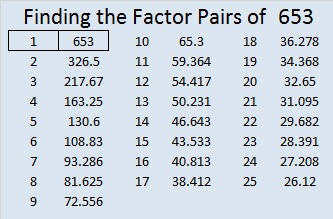# 653 and Level 4

When the last two digits of 653 are divided by 4, we get a remainder of 1 so there is an alternate way to tell if 653 is a prime number by first determining if it is the sum of any two perfect squares:

653 – 1 – 3 – 5 – 7 – 9 – 11 – 13 – 15 – 17 – 19 – 21 – 23 – 25 = 484 which is 22².

653 – 22² = 169 which is 13².

Thus 22² + 13² = 653, and 653 is the sum of two perfect squares.

From that mathematical statement we observe the following:

• 22 and 13 have no common prime factors, and 653 is odd.
• 653 is the hypotenuse of the primitive Pythagorean triple 315-572-653 which was calculated using 22² – 13², 2(22)(13), 22² + 13².
• The only primitive Pythagorean triple hypotenuses less than √653 are 5, 13, and 17.
• 653 obviously is not divisible by 5 or 13.
• If 653 is not divisible by 17, it is a prime number. 653 is not divisible by 17.

Print the puzzles or type the solution on this excel file: 12 Factors 2015-10-19

——————————————————————

• 653 is a prime number.
• Prime factorization: 653 is prime.
• The exponent of prime number 653 is 1. Adding 1 to that exponent we get (1 + 1) = 2. Therefore 653 has exactly 2 factors.
• Factors of 653: 1, 653
• Factor pairs: 653 = 1 x 653
• 653 has no square factors that allow its square root to be simplified. √653 ≈ 25.55386.How do we know that 653 is a prime number? If 653 were not a prime number, then it would be divisible by at least one prime number less than or equal to √653 ≈ 25.6. Since 653 cannot be divided evenly by 2, 3, 5, 7, 11, 13, 17, 19, or 23, we know that 653 is a prime number.

We also know that 653 is a prime number from the observations made at the beginning of this post.

—————————————————————————————————This site uses Akismet to reduce spam. Learn how your comment data is processed.